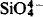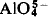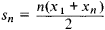# isomorphism

(redirected from List of nonisomorphic groups)
Also found in: Dictionary, Thesaurus, Medical.
Related to List of nonisomorphic groups: Abelian group

## isomorphism

(ī'səmôr`fĭzəm), of minerals, similarity of crystal structure between two or more distinct substances. Sodium nitrate and calcium sulfate are isomorphous, as are the sulfates of barium, strontium, and lead. Crystals of isomorphous substances are almost identical. The substances sometimes crystallize together in a solid solution. Isomorphous substances usually have similar chemical formulas, and the polarizability and ratio of anion and cation radii are generally comparable (see ionion,
atom or group of atoms having a net electric charge. Positive and Negative Electric Charges

A neutral atom or group of atoms becomes an ion by gaining or losing one or more electrons or protons.
). Isomorphism was discovered (c.1820) by Eilhard Mitscherlich, who stated the principle that isomorphous substances have similar chemical formulas; this principle was used by J. J. Berzelius in determining chemical formulas and combining weights. See polymorphismpolymorphism,
of minerals, property of crystallizing in two or more distinct forms. Calcium carbonate is dimorphous (two forms), crystallizing as calcite or aragonite. Titanium dioxide is trimorphous; its three forms are brookite, anatase (or octahedrite), and rutile.
; mineralmineral,
inorganic substance occurring in nature, having a characteristic and homogeneous chemical composition, definite physical properties, and, usually, a definite crystalline form. A few of the minerals (e.g.
; crystalcrystal,
a solid body bounded by natural plane faces that are the external expression of a regular internal arrangement of constituent atoms, molecules, or ions. The formation of a crystal by a substance passing from a gas or liquid to a solid state, or going out of solution (by
.
The following article is from The Great Soviet Encyclopedia (1979). It might be outdated or ideologically biased.

## Isomorphism

the ability of substances of similar chemical composition to crystallize in identical forms.

Isomorphism was first demonstrated by the German mineralogist E. Mitscherlich in 1819 with KH2PO4, KH2AsO4, and NH4H2Po4. It was quickly found that the first two of these substances form mixed crystals (solid solutions) with a single external shape in an arbitary P:As ratio; similar replacements with other pairs are quantitatively restricted. The former case corresponds to the concept of complete isomorphism; the latter, to that of incomplete, or restricted, isomorphism. If amounts of the replacing element are small, but essential for the prospector, mineralogist, and geochemist, one refers to endo-cryptic processes (according to A.E. Fersman’s definition, the replacement of nodes in crystal lattices by ions of the same sign but different properties and magnitudes). The necessity of relat-edness between elements replacing one another in isomorphism was originally taken as a necessity for purely chemical affinity, and the first series of isomorphous elements (V. I. Vernadskii) repeated the groups of Mendeleev’s periodic system, adding certain groups known from analytical chemistry, such as aluminum, chromium, and iron. However, it became clear, for example, that it is impossible to replace rubidium with sodium and that solid solutions of potassium and sodium compounds are vigorously decomposed at low temperatures (decomposition of potassium and sodium feldspars). The introduction of the notion of ionic radii was of decisive importance in explaining these phenomena, and the affinity of ionic radii became understood as one of the basic conditions of isomorphism (V. M. Goldschmidt, 1926). With whole-valence cations (Na1+, Mg2+, …, S6+…) the ionic radius decreases rapidly along the row in the Mendeleev system and increases sharpy along the vertical, by more than 10–15 percent (the experimental limit for possible isomorphism). Consequently, diagonally adjacent elements prove isomorphous (D. I. Mendeleev, A.E. Fersman); an example is the Na-Ca series (r-* = 0.98 A; rca = 1.02 A), as represented by the plagioclases, which make up more than 50 percent of the earth’s crust. This diagonal extends to the rare earth elements, and for a long time these elements were thought to be bivalent (only D.I. Mendeleev put them in Group III), precisely because of the continual isomorphous replacement by rare earth elements in calcium minerals. Other characteristic diagonal pairs are lithium-magnesium, molybdenum-rhenium, and beryllium-aluminum. However, in the long rows of the Mendeleev table (with 32 squares) the decrease in radius along the row goes so far that the radii of the cations of the same group flatten out; that is, for elements in the same group, divided vertically by lanthanide contraction, the isomorphism is extremely pronounced. This holds for the pairs barium-radium, zirconium-hafnium, and niobium-tantalum. But as close to one another as barium and tantalum, for example, may be, it is easier to separate them from one another than it is to separate either from titanium, with which they are connected by diagonal isomorphism. Thus, isovalent isomorphism is much less in evidence than heterovalent isomorphism (at any rate, qualitatively). The question arises of how the crystal structure compensates for valence change (for example, for its increase in Ca2+ — Na1+isomorphism). The solution to this question is simple when the element in the center of a diagonal is replaced by two neighbors on different sides, such as in 2Ca2+ ⇋ Na1+ + La3- and 2Ti4+ ⇋ Fe3+ + Nb5+.

Most frequently, compensation is by simultaneous heterovalent isomorphism in the reverse direction. In the plagioclases the replacement by Ca2+ of Na1+ is accompanied by a parallel replacement by Al3+ of Si4+: CaAl2Si2Os ⇋ NaAlSi3Og. The question arises of how this can take place when the radii of Si4+ (0.39 angstrom [Å]) and Al3+ (0.57 A) differ by 46 percent. A considerable difference between the radii is no hindrance in heterovalent isomorphism, since in the anionic, more negative, part of the compounds, it is not atoms but tetrahedral groups that replace one another—for example,and, where the effective Si—O and Al—O distances (1.72 and 1.90 Å) differ by only 9 percent. For example, lithium, in its more “cationic” form, with coordination number 6, replaces magnesium, in accordance with the diagonal law (in biotites); if it is among four oxygens, the lithium can replace the beryllium in beryl: [Li04] → [Be04]. The patterns that have been looked into concern basically cases of isomorphous substitutions between whole-valence ions of the noble gases type in compounds subject to the laws of elementary energetics (A. F. Kapustinskii’s formulas). The rules of isomorphism are different for the transition metals, which form compounds essentially of the covalent type, and tend to build around themselves 8-, 13–14-, and 18-electron groups by a donor-acceptor mechanism. Thus, with a pair of elements of identical radii, such as Zn2 and Fe2, one finds unilateral isomorphism. Zinc, in its primary compound, ZnS (sphalerite), allows the entry of as much as 20 percent iron; however, zinc is completely absent from FeS. The reason for this is that iron can be both four- and six-coordinate, whereas in sulfides zinc is always four-coordinated.

Isomorphism is widespread in nature. The complex chemistry of the composition of most minerals, especially in the silicate group, is explained by the extensive development of isomorphous replacements. Minerals of variable composition, forming continuous series, are examples of complete isomorphism; these minerals include the plagioclases, the scapolites, and the wolframites. The laws of isomorphous replacement explain the distribution of the rare elements that are present as impurities in rocks and ores. Thus, a considerable amount of yttrium and the rare earths is found in apatite, sphene, and fluorite, isomorphously replacing calcium; in magnetite, trivalent vanadium replaces iron oxide; and selenium replaces sulfur in pyrites. The theory of isomorphism is basic in studying the forms in which elements occur in rocks and the processes of the concentration and scattering of elements in the earth’s crust.

### REFERENCES

Fersman, A.E. Geokhimiia, 2nd ed., vol. 1. Leningrad, 1934.
Mendeleev, D.I. Soch., vol. 1. Leningrad, 1937.
Goldschmidt, V.M. Krista/lokhimiia.Lemngrad, 1937. (Translated from German.)
Sto let perioicheskogo zakona khimicheskikh elementov. Moscow, 1969.

N. V. Belov

## Isomorphism

one of the fundamental concepts of modern mathematics, which initially arose in algebra in connection with algebraic systems such as groups, rings, and fields but which proved to be extremely significant for the understanding of the structure and domain of possible applications of every branch of mathematics.

The concept of isomorphism applies to systems of objects on which operations or relations are defined. As a simple example of two isomorphic systems consider the system R of all real numbers under the operation of addition x = x1 + x2 and the system P of positive real numbers under the operation of multiplication y = y1y2. It turns out that the internal “layout” of these two systems of numbers is identical. To show this we map the system R onto the system P by associating to the number y = ax (a > 1) in P the number x in R. Then the product y = y1y2 of the numbers y1 = ax1 and y2 = ax, which correspond to x1 and x2, will correspond to the sum x = x1 + x2. The inverse mapping of p onto R is given by x = logay. From any proposition concerning the addition of numbers in the system R we can obtain a corresponding proposition concerning the multiplication of numbers in the system P. For example, since in R the sum

sn = X1, + X2 + ... + Xn

of the terms of an arithmetic progression is given by the formulait follows that in P the product

Pn = y1y2 ... yn

of the terms of the corresponding geometric progression is given by the formula(raising to the nth power in P corresponds to multiplication by n in R and extraction of the square root in P corresponds to division by two in R).

As regards their properties, isomorphic systems are essentially the same. From an abstract mathematical standpoint, such systems are indistinguishable. Any system of objects S’ that is isomorphic to a system 51 may be regarded as a “model” of 5 (modeling a system S by means of a system S ’), and the study of the properties of S may be reduced to the study of the properties of the “model” S’ of S.

The following is a general definition of the isomorphism system of objects such that each system has a number of relations and each relation involves a fixed number of objects. Let S and S’ be two given systems of objects. Let

Fk(x1,x2,...), k = 1,2, ... , n

be the relations on S and let

F’k(x1,x2, ... ), k = 1,2, ..., n

be the relations on S’. The systems S and S’, with their respective relations, are said to be isomorphic if there exists a one-to-one correspondence

x’ = ø(x) x = Ψ(x’)

between the elements of S and S” such that Fk (x1,x2, ... ) implies F’k (x1, x2,... ) and vice versa. The correspondence is said to be an isomorphic map, or an isomorphism. [In the example cited above, the relationF(x, x1, x2), where x = xx + x2, is defined on the system R, and the relation F’(y, y1 y2) where y = y1y y2, is defined on the system P; a one-to-one correspondence is given by the formulas y = ax and x = log ay]

The concept of isomorphism arose in group theory, where the fact that the study of the internal structure of two isomorphic systems of objects represents one rather than two problems was first understood.

The axioms of any mathematical theory determine the system of objects studied by the theory only up to isomorphism: a mathematical theory based on axioms that is applicable to one system of objects is always fully applicable to another. Therefore, every axiomatic mathematical theory allows not one but many “interpretations,” or “models.”

The concept of isomorphism includes, as a particular case, the concept of homeomorphism, which plays a fundamental role in topology.

A particular case of an isomorphism is an automorphism, which is a one-to-one mapping

x’ = ø(x) x = Ψ(x’)

of a system of objects with given relations Fk (x1, x2 ... ) onto itself such that Fk(xx, x2 ...) implies Fk(x’1, x’2 ...) and vice versa. This concept also arose in group theory but later proved significant in the most disparate branches of mathematics.

### REFERENCES

Kurosh, A.G. Kurs vysshei algebry, 3rd ed. Moscow-Leningrad, 1952.
Entsiklopediia elementarnoi matematiki, book 2. Edited by P.S. Alek-sandrov (et al.). Moscow-Leningrad, 1951.

## isomorphism

[¦ī·sə¦mȯr‚fiz·əm]
(mathematics)
A one to one function of an algebraic structure (for example, group, ring, module, vector space) onto another of the same type, preserving all algebraic relations; its inverse function behaves likewise.
(physical chemistry)
A condition present when an ion at high dilution is incorporated by mixed crystal formation into a precipitate, even though such formation would not be predicted on the basis of crystallographic and ionic radii; an example is coprecipitation of lead with potassium chloride.
(science and technology)
The quality or state of being identical or similar in form, shape, or structure, such as between organisms resulting from evolutionary convergence, or crystalline forms of similar composition.
McGraw-Hill Dictionary of Scientific & Technical Terms, 6E, Copyright © 2003 by The McGraw-Hill Companies, Inc.

## isomorphism

1. Biology similarity of form, as in different generations of the same life cycle
2. Chem the existence of two or more substances of different composition in a similar crystalline form
3. Maths a one-to-one correspondence between the elements of two or more sets, such as those of Arabic and Roman numerals, and between the sums or products of the elements of one of these sets and those of the equivalent elements of the other set or sets
Collins Discovery Encyclopedia, 1st edition © HarperCollins Publishers 2005

## isomorphism

(mathematics)
A bijective map between two objects which preserves, in both directions, any structure under consideration. Thus a `group isomorphism' preserves group structure; an order isomorphism (between posets) preserves the order relation, and so on. Usually it is clear from context what sort of isomorphism is intended.